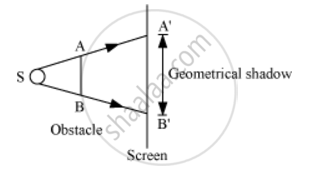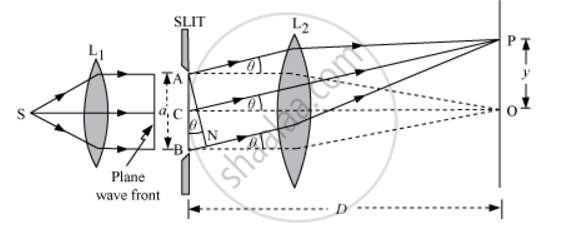Share

Describe Briefly How a Diffraction Pattern is Obtained on a Screen Due to a Single Narrow Slit Illuminated by a Monochromatic Source of Light. Hence Obtain the Conditions for the Angular Width of Secondary Maxima and Secondary Minima. - CBSE (Science) Class 12 - Physics

ConceptFraunhofer Diffraction Due to a Single Slit

Question

Describe briefly how a diffraction pattern is obtained on a screen due to a single narrow slit illuminated by a monochromatic source of light. Hence obtain the conditions for the angular width of secondary maxima and secondary minima.

Solution

The phenomenon of bending of light round the sharp corners of an obstacle and spreading into the regions of the geometrical shadow is called diffraction.Expression For Fringe WidthConsider a parallel beam of light from a lens falling on a slit AB. As diffraction occurs, the pattern is focused on the screen XY with the help of lens L2. We will obtain a diffraction pattern that is a central maximum at the centre O flanked by a number of dark and bright fringes called secondary maxima and minima.

Central Maximum − Each point on the plane wave front AB sends out secondary wavelets in all directions. The waves from points equidistant from the centre C lying on the upper and lower half reach point O with zero path difference and hence, reinforce each other, producing maximum intensity at point O.

Positions and Widths of Secondary Maxima and Minima

Consider a point P on the screen at which wavelets travelling in a direction making angle θ with CO are brought to focus by the lens. The wavelets from points A and B will have a path difference equal to BN.

From the right-angled ΔANB, we have:

BN = AB sinθ

BN = a sinθ …(i)

Suppose BN = λ and θ = θ1

Then, the above equation gives

λ = a sin θ1

sintheta_1=lambda/a

Such a point on the screen will be the position of first secondary minimum

If BN = 2λ and θ= θ2, then

2λ = a sin θ2

sin_2=(2lambda)/a

Such a point on the screen will be the position of second secondary minimum.

In general, for nth minimum at point P,

sintheta_n=(nlambda)/a

If yn is the distance of the nth minimum from the centre of the screen, from right-angled ΔCOP, we have:

tantheta_n=(OP)/(CO)

tantheta_n=y_n/D

In case θn is small, sin θn ≈ tan θn

∴ Equations (iv) and (v) give

y_n/D=(nlambda)/a

y_n=(nDlambda)/a

Width of the secondary maximum,

beta=y_n-y_(n-1)=(nDlambda)/a-((n-1)Dlambda)/a

beta=(Dlambda)/a ...(vi)

∴ β is independent of n, all the secondary maxima are of the same width β.

If BN=(3lambda)/2and theta=theta_1^', from equation (i), we have:

sin_1^'=(3lambda)/(2a)

Such a point on the screen will be the position of the first secondary maximum.

Corresponding to path difference,

BN=(5lambda)/2and theta=theta_2^'the second secondary maximum is produced

In general, for the nth maximum at point P,

sin_n^'=((2n+1)lambda)/(2a)

If y_n^'is the distance of nth maximum from the centre of the screen, then the angular position of the nth maximum is given by

tantheta_n^'=y_n^'/D

In case theta_n^'is small

sintheta_n^' ~~tantheta_n^'

:.y_n^'=((2n+1)Dlambda)/(2a)

Width of the secondary minimum,

β' = y'n  y'n1 (nDlambda)/a-((n-1)Dlambda)/a

beta'=(Dlambda)/a

Since β' is independent of n, all the secondary minima are of the same width β'

Is there an error in this question or solution?
Solution Describe Briefly How a Diffraction Pattern is Obtained on a Screen Due to a Single Narrow Slit Illuminated by a Monochromatic Source of Light. Hence Obtain the Conditions for the Angular Width of Secondary Maxima and Secondary Minima. Concept: Fraunhofer Diffraction Due to a Single Slit.
S